## Azdren's Excel Reference Sheet

last updated: 11/20/22

Disclaimer: This is a work-in-progress, there may be errors. If you find a flaw, please let me know via email.

Everybody knows about Excel. My concern is that not a lot of people know about the power and utility of Excel. That is why I am creating this reference sheet. It wasn’t until I was a fourth year graduate student that I learned many of these tools. I regret not knowing about these tools before, and I don’t want other graduate students to feel the same.

The reference sheet below is just a scratch on the surface of what Excel can do. That being said, I hope that it is useful as a reference, and that you may find something new that you did not know about before.

Note: the green highlighted text = excel code. Every different color is a different set of codes.

=IFERROR(F6>0,”No”)

Returns a ‘true’ value if the value of F6 is

greater than 0, a “No” otherwise.

=IF(F6>0,”YES”,”NO”)

– Returns a “YES” if the value of F6 is
greater than zero, and a “No” otherwise.

=IF(F6>93,”A”,IF(F6>90,”A-“,….”F”)

IF functions can be iterated a multiple of times

in a nested method, either in the “True” return, or the “false” return. The
above is an example of a nested IF statement, where the nested statement is in
the ‘false’ return. This function would return an “A” if the value of F6 is
greater than 93, but if the value is false, it will calculate the next IF
function of F6>90. If the second if function, the return will be an “A-“, otherwise
it will go to the next ‘false’ statement, and so on.

=SEARCH(“North America”,F6)

Returns a ‘true’ value if the string “North

America” is found anywhere in the cell F6

=IF(SEARCH(“North America”,F6),1,0)

Returns a “1” if the text is found in F6, a “0”

otherwise.

=IFERROR(IF(SEARCH(“North America”,A1)>0,1,0),0)

In addition to the above, the first IFERROR

function will return a “0” when the value in the cell A1 is invalid.

=ABS(“-3 1/2”)

returns “3.5”, or the absolute value of “-3

1/2″

=LOWER(“hELlO”)

returns all lowercase letters, “hello”

=SUBSTITUTE(D3,” “,””)

if there are spaces in the text in D3, it will

return without spaces, because it is substituting “ “, which is a space, for
“”, which is empty.

In other words, if the text is “hello world”,

this function will return “helloworld”

=SUBSTITUTE(SUBSTITUTE(D3,”-“,””),” “,””)

This “substitute” within a “substitute” rule

will change “ “ to “”, but also “-“ to “”.

In other words, the above function will convert

this: “V – 1” to “v1”

=TRIM(D3)

Removes multiple spaces from the text in D3

For example, “Hello          world. My name      is

Azdren” turns into “Hello world. My name is Azdren”

=TRIM(SUBSTITUTE(D3,”-“,””))

This uses the trim function, while also removing

every dash (“-“) symbol from the text.

For example, “Hello     world -.” becomes “Hello world .”

=LEN(D4)

This returns the length of a string.

For example, if a string is “Hello”, the return

is 5.

Or, the string “hello         world.” returns the value 20.

=LEN(TRIM(D5))

The ‘Len’ function can be used in conjunction

with other functions, such as with ‘Trim’, to remove an additional step of
having to Trim the text before finding the length.

For example, Len(Trim(D5) where D5 = “hello         world.” Returns a value of 12. Recall

that the same string above returned a value of 20.

=FIND(“V”, D6)

Suppose the string D6 = “V1 – 11 V2 – 8 V3 – 9”.

The return for Find “V” in D6 is 1, since the first character in that
string is “V”.

=FIND(“V”&VALUE(C6),D6)

Suppose we want to find a particular value in

the string “V1 – 11 V2 – 8 V3 – 9”, where V1, V2, and V3 denote different
values. First, we would want to find the character location of the values we
are looking for. For this, we combine several functions. The & symbol joins
text. Furthermore, the VALUE(C6)
function returns the value of that cell. In this case, C6 denotes which V
number we want to extract. The value of “V”&VALUE(C6) would return “V3”.
Therefore,  if C6 = 3, and if we use the
above function, the return will be 16, since the character count up to
“V3” (including spaces) is 16.

If we know that V3 is the last value in the

string, we can use the RIGHT
function to retrieve the data in the string for V3. If V3 is not the last
value, then we can retrieve the data by using a FIND function for the value of
V4, and retrieve the information using a MID function.

=FIND(“V”&VALUE(C6),SUBSTITUTE(D6,” “,””))

In addition to the above, this function returns

the location value of the characters without counting any spaces.

=FIND(“V”&VALUE(C6),TRIM(D6))

Similar to the previous function, this

Supposed out string in cell C6 is:

“V1 – 11 V2 – 8 V3 – 9”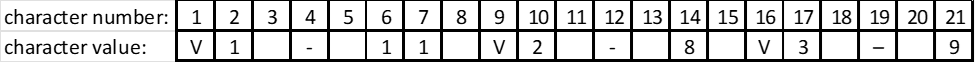=LEFT(C6, 4)

This returns a string from the string in C6, beginning from the left end of the string, counting 4 characters.

For example, the above function would return “V1 –“ since this is four characters long (including the spaces).

=MID(C6, 1, 4)

This function returns a string in the middle of another string. The first value “C6” is the string location, while the second value is “1” denotes where the character count should begin. The third value “4” is specifying how many characters to return from the original string.

For example, the above function would return “V1 –“ again, since just like LEFT, it begins at the first character and counts 4.

But if we specified MID(C6, 5, 5), this will return “ 11 V”, which includes a space in the beginning of the string.

=RIGHT(C6,7)

This returns a string of 7 characters in length from the right of another string, in cell C6.

For example, the above function would return “ V3 – 9”, since, including the spaces, this is seven characters.

The above three functions, in conjunction with other functions such as FIND and LEN, will allow you to capture a large number of strings from other string values in your data cleaning process.

For example, consider the following function:

=RIGHT(C6,LEN(C6)FIND(“V3”,C6))

This would return “3 – 9”, because LEN(C6) is 21, and FIND(“V3”,C6) would return 16, and the difference between the two terms is 5, which means that the RIGHT function will return five characters starting from the right, up until the Beginning of “V3”.

Because of the way Excel counts characters, we need to add a “-4” to the function (to shorten the length of the return, so that the return itself just gives the value of V3, so it would look like this: =RIGHT(C6,LEN(C6)-FIND(“V3”,C6)-4)

=TEXTAFTER(C6,” – “)

Returns the test that is after the symbol “ – “

=CLEAN(C6)

Removes low-level computer code that can’t be displayed, such as strange characters, line breaks.

=VLOOKUP(C6,\$D\$6:\$G\$20,3,FALSE)

This would return the value in the third column (3) in the fixed table array \$D\$6:\$G\$20 (the ‘\$’ fixes the values in the range of the table) based on an exact match (FALSE = exact match) with the value in C6.

=PROPER(C6)

Capitalizes the beginning of each word

=UPPER(C6)

Makes all text uppercase

=LOWER(C6)

Makes all text lowercase

=COUNTIF(C6:C12,”P”)

Counts the total number of times the cell has a value of “P” in the given range.

## To return a “1” if a string is found within a cell:

=IF(ISNUMBER(SEARCH(“blahblah”, C6)),1,0)

– Where “blahblah” is the string you are searching for within  C6.

– The “ISNUMBER” command returns a true value if it finds the text. Otherwise, SEARCH will tell you the character number where the string begins within cell C6

## Other tips:

–          Select cells then hold SHIFT while moving them.

–          CTRL + ;                Enters current date.

–          CTRL + SHIFT + ;                 Enters current time.

# Macros in Excel

Disclosure: This is a very basic implementation of Macros in Excel. But it should give you a sense of what is possible.

·         Microsoft has made it very easy to record Macros. Recording Macros basically records a series of actions you take within an Excel document that you may want to repeat multiple times.

·         For example, you can record a Macro of changing the font of the whole sheet, or only of a particular cell; typing in a formula and creating a new value; deleting and creating cells. For me, it was very helpful in formatting a report with a separate sheet for each county of WA. A process that may have taken me several workdays only took me a few hours because instead of formatting over 30 sheets, I only had to format one.

·         From my understanding (I know I am wrong here, I don’t know how to format things differently), the Macros function somewhat blindly so that the sheets that need to be formatted should all be prepared in the same layout. For example, the data begins in cell B2 and ends in B10.

·         The actual Macros themselves can be saved, but the Excel document needs to be saved

·         Break up the Macros tasks into smaller Macros. Don’t save one Macro for the entire task. In my experience, doing a task in three parts is exponentially faster. It uses fewer resources than doing the same tasks in one macro. The allocation of computational resources is not proportional for some reason.

#### To record your first Macro

1. To begin, click on the “View” tab on the top.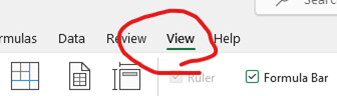2. Locate the “Macros” tool to the right of the page. Click on the bottom half of the tool so that a drop-down menu appears.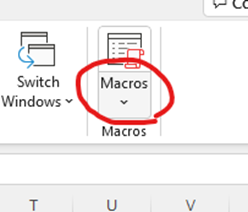3. Click on “Record Macros…”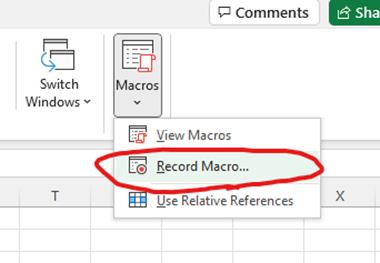4. Name your Macro, assign a shortcut key, and add a description. Warning: You can create shortcut keys, but do not create a shortcut key that is already in existence (such as ctrl+’s’ or ctrl+’z’). Excel will allow you to create these shortcuts, but then will likely crash when trying to use the shortcut.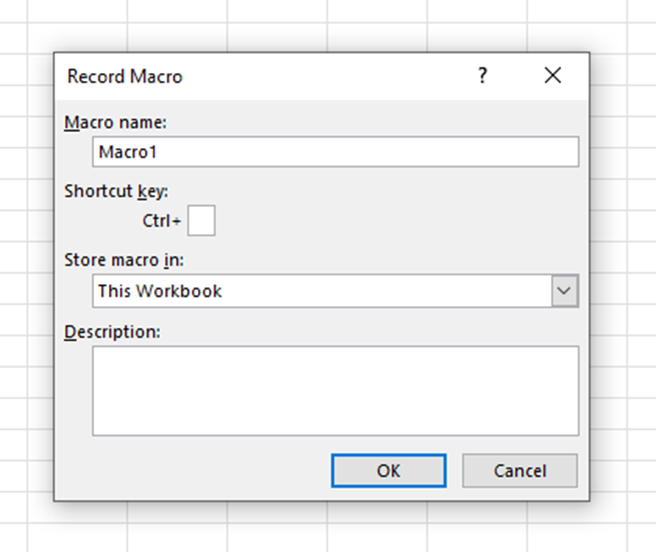5. Begin making the changes to the Excel spreadsheet that you want to record. Once you are done, go back to “View,” click the drop-down menu for “Macros,” and click on “Stop Recording.”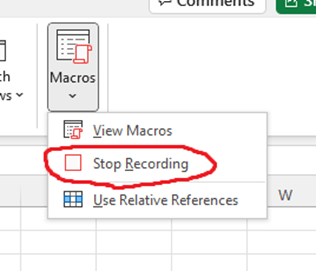6. To use the previously recorded Macro, click on “View,” then click directly on “Macros,” then select the Macro you want to use, and click “Run.”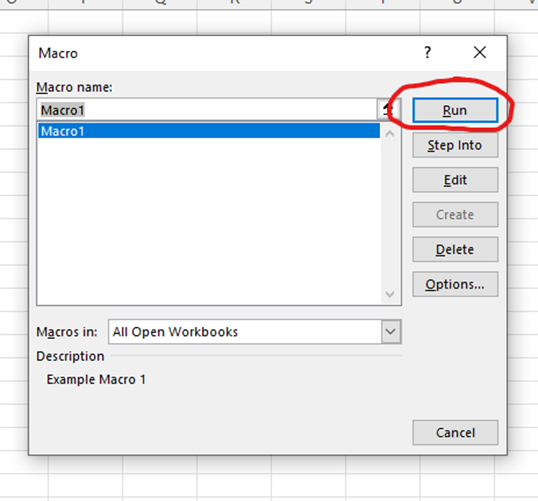7. If you want to save the Macros (you don’t have to), you need to save them as a special “Excel Macro-Enabled Workbook.”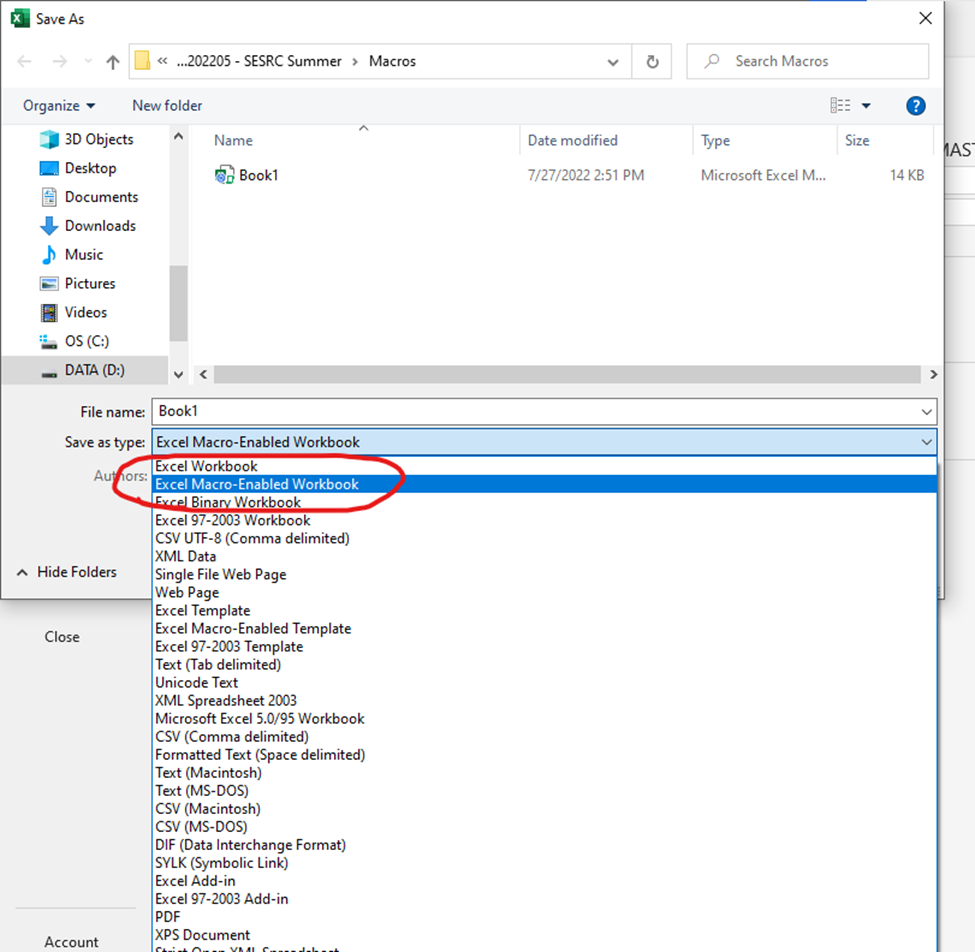8. Macros may be disabled to run from a saved Excel file (for a good reason), so you must enable Macros to run manually. Go to “File,” then “Options,” then click on “Trust Center,” then “Trust Center Settings.”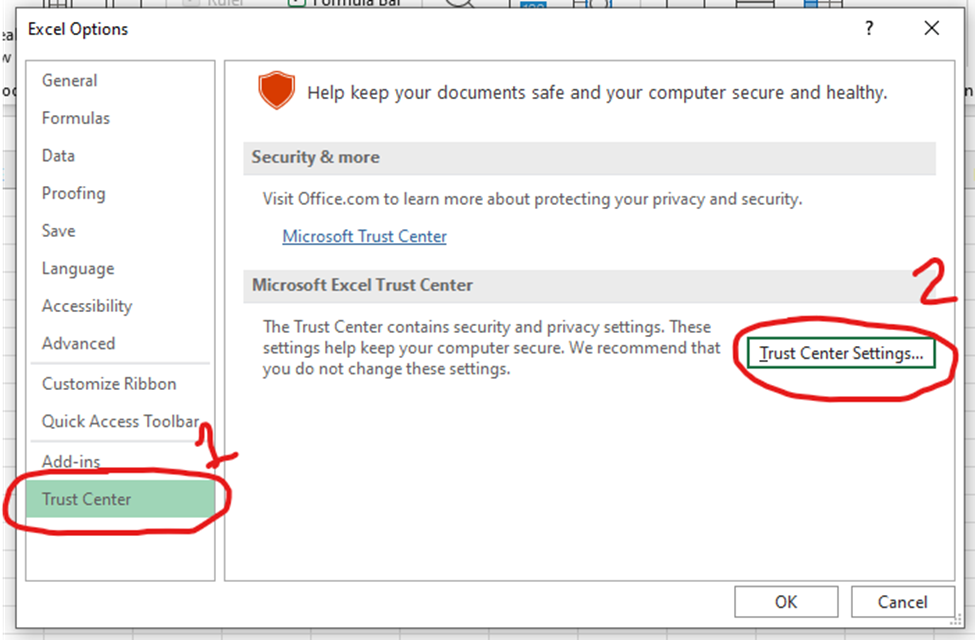Then “Macro Settings,” and click on “Enable VBA macros.”